Thermodynamics and Propulsion
Subsections

6.4 Brayton Cycle in-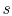Coordinates

The Brayton cycle has two reversible adiabatic (i.e., isentropic) legs and two reversible, constant pressure heat exchange legs. The former are vertical, but we need to define the shape of the latter. For an ideal gas, changes in specific enthalpy are related to changes in temperature by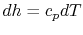, so the shape of the cycle in an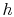-plane is the same as in a-plane, with a scale factor of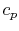between the two. This suggests that a place to start is with the combined first and second law, which relates changes in enthalpy, entropy, and pressure: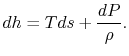On constant pressure curves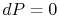and. The quantity desired is the derivative of temperature,, with respect to entropy,, at constant pressure: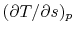. From the combined first and second law, and the relation between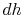and, this is(6..2)

The derivative is the slope of the constant pressure legs of the Brayton cycle on a-plane. For a given ideal gas (specific) the slope is positive and increases as.

We can also plot the Brayton cycle in an-plane. This has advantages because changes in enthalpy directly show the work of the compressor and turbine and the heat added and rejected. The slope of the constant pressure legs in the-plane is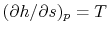.

Note that the similarity in the shapes of the cycles in-and-planes is true for ideal gases only. As we will see when we examine two-phase cycles, the shapes look quite different in these two planes when the medium is not an ideal gas.Plotting the cycle in-coordinates also allows another way to address the evaluation of the Brayton cycle efficiency which gives insight into the relations between Carnot cycle efficiency and efficiency of other cycles. As shown in Figure 6.4, we can break up the Brayton cycle into many small Carnot cycles. The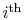'' Carnot cycle has an efficiency ofwhere the indicated lower temperature is the heat rejection temperature for that elementary cycle and the higher temperature is the heat absorption temperature for that cycle. The upper and lower curves of the Brayton cycle, however, have constant pressure. All of the elementary Carnot cycles therefore have the same pressure ratio:From the isentropic relations for an ideal gas, we know that pressure ratio,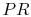, and temperature ratio,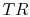, are related by:.

The temperature ratios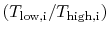of any elementary cycle i'' are therefore the same and each of the elementary cycles has the same thermal efficiency. We only need to find the temperature ratio across any one of the cycles to find what the efficiency is. We know that the temperature ratio of the first elementary cycle is the ratio of compressor exit temperature to engine entry (atmospheric for an aircraft engine) temperature,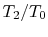in Figure 6.4. If the efficiency of all the elementary cycles has this value, the efficiency of the overall Brayton cycle (which is composed of the elementary cycles) must also have this value. Thus, as previously,A benefit of this view of efficiency is that it allows us a way to comment on the efficiency of any thermodynamic cycle. Consider the cycle shown in Figure 6.5, which operates between some maximum and minimum temperatures. We can break it up into small Carnot cycles and evaluate the efficiency of each. It can be seen that the efficiency of any of the small cycles drawn will be less than the efficiency of a Carnot cycle betweenand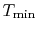. This graphical argument shows that the efficiency of any other thermodynamic cycle operating between these maximum and minimum temperatures has an efficiency less than that of a Carnot cycle.

Muddy Points

If there is an ideal efficiency for all cycles, is there a maximum work or maximum power for all cycles? (MP 6.7)

6.4.1 Net work per unit mass flow in a Brayton cycle

In Section 3.7.1 we found the net work of a Brayton cycle in terms of heat transfer. Now that we have defined entropy, we can reexamine the net work using an enthalpy-entropy (-) diagram, Figure 6.6. The net mechanical work of the cycle is given by:where, by the first law,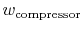If kinetic energy changes across the compressor and turbine are neglected, the temperature ratio,, across the compressor and turbine is related to the enthalpy changes: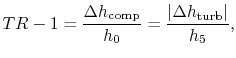The net work is thus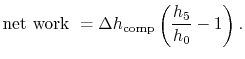The turbine work is greater than the work needed to drive the compressor, as is evident on the (-) diagram.Douglas Quattrochi 2006-08-06